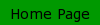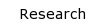# Riccardo Fazio Reprints###An Iterative Transformation Method for a Similarity Boundary Layer Model. Preprint, 2023.Free Boundary Formulation for Boundary Value Problems on Semi-Infinite Intervals: An up to Date Review. Submitted to: Computer \& Fluids, 2023.A Non-Iterative Transformation Method Applied to Boundary-Layer Flows of Non-Newtonian Fluids. Submitted to: J. Comput. Sci., 2023.Numerical Transformation Mehtods for a Moving-Wall Boundary Layer Flow of a Rarefied Gas Free Stream over a Moving Flat Plate. Submitted to: Comp. Math. Appl., 2023.Free Boundary Formulation of two Extended Blasius Problems. Int. J. Non-Linear Mech., 153 (2023) 104423.A Non-Iterative Transformation Method for Boundary-Layer with Power-Law Viscosity for non-Newtonian Fluids. Calcolo, 59, 43, 2022.A Front Fixing Implicit Finite Difference Method for the American Put Option Model. Joint work with: A. Jannelli and A. Insana. Math. Comput. Appl., 1, 0, 2021.A Non-Standard Finite Difference Scheme for Magneto-Hydro-Dynamics Boundary Layer Flow of an Incompressible Fluid Past a Flat Plate. Joint work with A. Jannelli, Math. Comput. Appl., 26, 22, 2021.Existence and Uniqueness of BVPs Defined on Infinite Intervals: Insight from the Iterative Transformation Method. Math. Comput. Appl., 26, 18, 2021.Perpetual American Put Option: an Error Estimator for a Non-Standard Finite Difference Scheme. Appl. Math. E-Notes, 21, 282-290, 2021.A Non-Iterative Transformation Method for an Extended Blasius Problem. Math. Meth. Appl. Sci., 44, 1996-2001, 2021.Scaling Invariance and Numerical Transformation Methods: A Unifying Framework. Appl Engin. Sci., 4, 100024, 2020.OCTAVE per l'Economia e la Finanza. TGBook Ed., Sandrigo, 2020.American Put Option: Richardson's extrapolation and a Posteriori Error Estimator for a Front-Fixing Finite Difference Scheme. Appl. Math. E-Notes, 20,445-457, 2020.The Iterative Transformation Method. Int. J. Nonlinear Mech., 116, 181-194, 2019.The Non-Iterative Transformation Method. Int. J. Nonlinear Mech., 114, 41-48, 2019.Numerical study on gas flow through a micro-nano porous medium based on finite difference schemes on quasi-uniform grids. Joint work with A. Jannelli and T. Rotondo. Int. J. Non-Linear Mech., 105, 186-191, 2018.Two finite difference methods for a nonlinear BVP arising in physical oceanography. Joint work with A. Jannelli. AAPP | Atti della Accademia Peloritana dei Pericolanti, 96, A3, 2018.BVPs on infinite intervals: A test problem, a nonstandard finite difference scheme and a posteriori error estimator. Joint work with A. Jannelli, Math. Meth. Appl. Sci., 40, 6285-6294, 2017.Well-posed initial conditions and numerical methods for one-dimensional models of liquid dynamics in a horizontal capillary. Joint work with A. Jannelli. Comput. Appl. Math., 36, 903-913, 2017.Front fixing finite difference schemes for American put options model. Joint work with A. Insana and A. Jannelli, International Conference of Numerical Analysis and Applied Mathematics 2015 (ICNAAM 2015) AIP Conf. Proc. 1738, 480123-1--480123-4, 2016.A Non-Iterative Transformation Method for Blasius Equation with Moving Wall or Surface Gasification, Int. J. Non-Linear Mech., 78, 156-159, 2016.The Iterative Transformation Method for the Sakiadis Problem, Comput. & Fluids, 106, 196-200, 2015.Free Boundary Formulation for BVPs on a Semi-infinite Interval and Non-iterative Transformation Methods. Acta Appl. Math., 140, 27-42, 2015.A Posteriori Error Estimator for a Front-Fixing Finite Difference Scheme for American Options. WCE2015, London June 30 - July 3, 2015.Metodi Numerici per Problemi ai Valori Iniziali, TGBook Ed., Sandrigo, 2014.Elementi di Analisi Numerica, TG Book ed., 2014.Finite Difference Schemes on Quasi-Uniform Grids for BVPs on Infinite Intervals. Joint work with A. Jannelli. J. Comput. Appl. Math., 269, 14-23, 2014.A Non-Iterative Transformation Method for Newton's Free Boundary Problem. Int. J. Non-Linear Mech., 59, 23-27, 2014.An Analytical and Numerical Study of Liquid Dynamics in a 1D Capillary under Entrapped Gas Action. Joint work with S. Iacono. Math. Meth. Appl. Sci., 37, 2923-2933, 2014.Quasi-uniform Grids and Ad Hoc Finite Difference Schemes for BVPs on Infinite Intervals. Joint work with A. Jannelli. ENUMATH 2013.Blasius Problem and Falkner-Skan model: Toepfer's Algorithm and its Extension. Computers Fluids, 73, 202-209, 2013.Scaling Invariance and the Iterative Transformation Method for a Class of Parabolic Moving Boundary Problems. Int. J. Non-Linear Mech., 50, 136-140, 2013.Extended Scaling Invariance of One-dimensional Models of Liquid Dynamics in a Horizontal Capillary. Joint work with S. Iacono, A. Jannelli, G. Cavaccini, and V. Pianese. Math. Meth. Appl. Sci., 35, 935-942, 2012.A Central Schemes and Second Order Boundary Conditions for 1D Interface and Piston Problems in Lagrangian Coordinates. Joint work with G. Russo. Commun. Comput. Phys., 8, 797-822, 2010.An Instance of Failure for the MATLAB Explicit ODE45 Solver. Proceedings ICAEM 2010. London, June 30 - July 2, 2010.On the Moving Boundary Formulation for Parabolic Problems on Unbounded Domains. Joint work with S. Iacono. Int. J. Comput. Math., 87, 186-198, 2010.On the Equivalence of Non-Iterative Transformation Methods Based on Scaling and Spiral Groups. Joint work with S. Iacono. Math. Meth. Appl. Sci., 30, 585-591, 2010.One-dimensional Mathematical and Numerical Modeling of Liquids Dynamics in a Horizontal Capillary. Joint work with G. Cavaccini, V. Pianese, S. Iacono, and A. Jannelli. J. Comput. Meth. Sci. Eng., 9, 3-16, 2009.Numerical Transformation Methods: Blasius Problem and its Variants. Appl. Math. Comput., 215, 1513-1521, 2009.Second Order Positive Schemes by means of Flux Limiters for the Advection Equation. Joint work with A. Jannelli. IAENG Int. J. Appl. Math., 2009.Pseudo-Spectral Methods for Linear Advection and Dispersive Problems. Joint work with S. Iacono. IAENG Int. J. Appl. Math., 2009.Second Order Numerical Operator Splitting for 3D Advection-Diffusion-Reaction Models. Joint work with A. Jannelli. Proceedings ENUMATH 2009.Liquid Penetrant Testing: Industrial Process. Joint work with G. Caturano, G. Cavaccini, A. Ciliberto, and V. Pianese, Communications to SIMAI Congress, 2009.Liquid Dynamics in a Horizontal Capillary: Extended Similarity Analysis. Joint work with S. Iacono, A. Jannelli, G. Cavaccini, and V. Pianese, to appear: Communications to SIMAI Congress, 2009.Ill and Well-Posed One-Dimensional Models of Liquid Dynamics in a Horizontal Capillary. Joint work with A. Jannelli, Communications to SIMAI Congress, 2009.On Translation Groups and Non-iterative Transformation Methods. Joint work with S. Iacono, Communications to SIMAI Congress, 2009.Transformation Methods for the Blasius Problem and its Recent Variants. Proceedings ICAEM 2008. London, July 2-4, 2008.Scaling and Spiral Equivalence from a Numerical Viewpoint. Joint work with S. Iacono. Proceedings ICAEM 2008. London, July 2-4, 2008.Numerical Scaling Invariance Applied to the van der Pol Model . Acta Appl. Math., 104, 107-114, 2008.A Two Immiscible Liquids Penetration Model for Surface-Driven Capillary Flows. Joint work with S. Iacono, A. Jannelli, G. Cavaccini, and V. Pianese. Presented at the ICIAM 2007.On the Moving Boundary Formulation for Parabolic Problems on Unbounded Domains. Joint work with S. Iacono. Presented at the ICIAM 2007.Invited talk at WASCOM07 Congress, Scicli, June 30 -- July 7, 2007, joint work with A. Jannelli.Local Error Reduction for First Order Implicit Pseudo-Spectral Methods Applied to Linear Advection Models. Joint work with Alessandra Jannelli and Salvatore Iacono.Mathematical and Numerical Modeling of Liquids Dynamics in a Horizontal Capillary. Joint work with G. Cavaccini, V. Pianese, S. Iacono, and A. Jannelli. Presented at the ICCMSE 2006.Mathematical and Numerical Modeling for a Bio-Chemical Aquarium. Joint work with A. Jannelli. Appl. Math. Comp., 174, 1370-1383, 2006Implicit Pseudo-Spectral Methods for Dispersive and Wave Propagation Problems. Joint work with A. Jannelli. Communications to SIMAI Congress, 2006.Adaptive Stiff Solvers at Low Accuracy and Complexity. Joint work with A. Jannelli, J. Comput. Appl. Math., 191, 246-258, 2006.Numerical Analysis for Advection-Diffusion-Reaction Models in 3D. Joint work with A. Jannelli, Poster presented at NAC2005, Numerical Analysis: the State of the Art, Cosenza, May 21-25, 2005.Moving-Mesh Methods for One-dimensional Hyperbolic Problems Using CLAWPACK. Joint work with Randy J. LeVeque. Comp. Math. Appl., 45, 273-298, 2003A Free Boundary Approach and Keller's Box Scheme for Boundary Value Problems on Infinite Intervals. Int. J. Comput. Math., 80, 1549-1560, 2003.Stiffness in Numerical Initial Value Problems: A and L-Stability of Numerical Methods Int. J. Math. Ed. Sci. Tech., 32, 752-760, 2001. MATLAB files available: ALstab.zipThe Iterative Transformation Method: Numerical Solution of One-Dimensional Parabolic Moving Boundary Problems. 78, 213-223, Int. J. Comput. Math., 2001.Comparison of Two Conservative Schemes for Hyperbolic Interface Problems. Presented at the ENUMATH 2001 (Fourth European Conference on Numerical Mathematics), Ischia, 23-28 July 2001Similarity and Numerical Analysis of a Singular Moving Boundary Hyperbolic Problem. Presented at the 11th ECMI (the European Consortium for Mathematics in Industry) Conference, Torre Normanna -- Palermo, 26-30 September 2000.A Survey on Free Boundary Identification of the Truncated Boundary in Numerical BVPs on Infinite Intervals. Presented at the ICCAM 2000 Conference, Leuven, 17-21 July 2000. J. Comput. Appl. Math., 140, 331-344, 2002.A 3D Mathematical Model for the Prediction of Mucilage Dynamics. Joint work with A. Jannelli and D. Ambrosi. Presented at the AMIF (Applied Mathematics for Industrial) Flows 2nd Inter. Conference, Il Ciocco, 12-14 October 2000.A Lagrangian Central Scheme for Multi-Fluid Flows. Joint work with G. Russo. Presented at the Eighth International Conference on Hyperbolic Problems, Teory, Numerics, Applications, Magdeburg, 28 Febbraio - 3 Marzo, 2000.Numerical Applications of the Scaling Concept. Acta Appl. Math., 55, 1-25, 1999. For the Bibliography print the sc-gita.pdf file and use the BIB files: sc-gita.bibsc-gita2.bib right click and save as ...A Similarity Approach to the Numerical Solution of Free Boundary Problems. SIAM Rev., 40, 616-635, 1998A Numerical Test for the Existence and Uniqueness of Free Boundary Problems. Appl. Anal., 66, 89-100, 1997.A Novel Approach to the Numerical Solution of Boundary Value Problems on Infinite Intervals. SIAM J. Numer. Anal., 33, 1473-1483, 1996.The Falkner-Skan Equation: Numerical Solutions within Group Invariance Theory. Calcolo, 31, 115-124, 1994.An Implicit Difference Scheme for a Moving Boundary Hyperbolic Problem. Joint work with David J. Evans, Appl. Numer. Math., 12, 485-496, 1993.A Moving Boundary Hyperbolic Problem for a Stress Impact in a Bar of Rate-Type Materials. Wave Motion, 16, 299-305, 1992.Numerical Length Estimation for Tubular Flow Reactors. J. Comput. Appl. Math., 41, 313-321, 1992.The Blasius Problem Formulated as a Free Boundary Value Problem. Acta Mech, 95, 1-7, 1992.The Iterative Transformation Method and Length Estimation for Tubular Flow Reactors. Appl. Math. Comput., 42, 105-110, 1991.Normal Variables Transformation Methods Applied to Free Boundary Value Problems. Int. J. Comput. Math., 37, 189-199, 1990.Similarity and Numerical Analysis for Free Boundary Value Problems. Joint work with David J. Evans, Int. J. Comput. Math., 31, 215-220, 1990.A Nonlinear Hyperbolic Free Boundary Value Problem. Acta Mech., 81, 221-226, 1990.This is an experimental page. Comments, to Riccardo Fazio, will be most welcome.